# Data visualisation using cluster analysis

Cluster analysis serves as an extension to qualitative data representation through data visualisation. It is an exploratory technique for visualising patterns in a study by grouping sources or nodes. Cluster analysis helps understand the correlation between elements of interview responses. The correlation is visible through word similarity, coding similarity and attribute similarity. Visual representation of this correlation is popular. To perform Cluster analysis in Nvivo:

1. Click on ‘Explore’
2. Click on ‘Cluster Analysis’

A dialogue box will appear (figure below).Figure 1: Step 1 for performing cluster analysis

Select either ‘Sources’ or ‘Nodes’. Then click on ‘Next’.

The case research uses nodes for performing cluster analysis. A new window will appear. Click on ‘Select’ and then ‘Nodes’(figure below).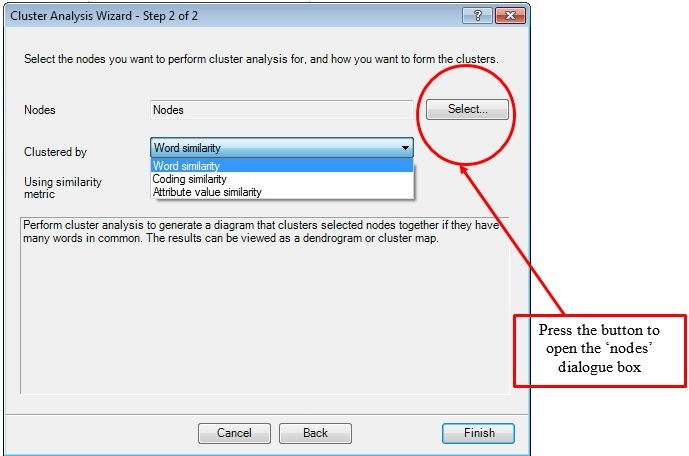Figure 2: Step 2 for performing cluster analysis

A dialogue box will appear (figure below). Here select all the nodes to include in cluster analysis. In the case research, select all the nodes and click on ‘OK’.Figure 3: Selecting items for cluster analysis

Next, select the appropriate ‘Clustered by’ option. This is based on three types of similarities. These are word similarity, coding similarity and attribute similarity. Choose ‘Word Similarity’ to show the similarity of words in selected nodes (figure below).

## Word similarity for cluster analysis in NvivoFigure 4: Cluster analysis using word similarity

Next select a ‘similarity metric’ (figure below). There are three types of similarity matrices: Jaccard’s Coefficient, Correlation Coefficient and Sorensen Coefficient. The current research uses correlation coefficient as the similarity metric. Then click on ‘Finish’.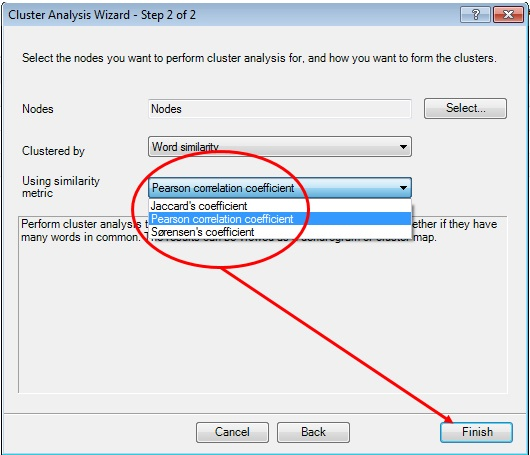Figure 5: Cluster analysis using Pearson Correlation Coefficient

A node ‘Cluster by Word Similarity’ will appear. Representation of ‘Cluster’ happens through two options; ‘Diagram’ and ‘Summary’ (figure below). The below figure is a diagram view.Figure 6: Results using word similarity

## Interpretation of diagram view

to interpret diagram view of cluster analysis by word similarity, export the diagram (figure below). Interconnected nodes are grouped together. For instance, curriculum, student’s participation and student’s performance are connected to each other. But they are not even remotely connected with other nodes like school management contribution, and preference for teaching. Nodes broadly show pattern between these two groups.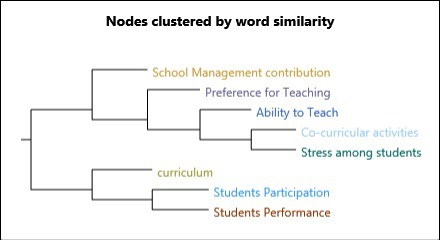Figure 7: Exported results

## Interpretation of summary view

The summary view of cluster analysis is a list of Pearson correlation coefficient between nodes (figure below). Its interpretation is similar to the statistical interpretation of correlation coefficient. Figure below shows a weak correlation among nodes as none of the values are greater than/ equal to .50.Figure 8: Summary view of cluster analysis results

One can also export summary view of cluster analysis in the form of excel sheet.

## Coding similarity

Cluster analysis through coding similarity is based on theme nodes. Two themes nodes may be coded with similar type of information. In the case research, student’s participation and student’s performance can have similar codes as their responses were similar. Manual coding does not allow dual coding of content in two nodes. Therefore coding similarity is enabled only in cases with auto-coding. The figure below represents coding similarity.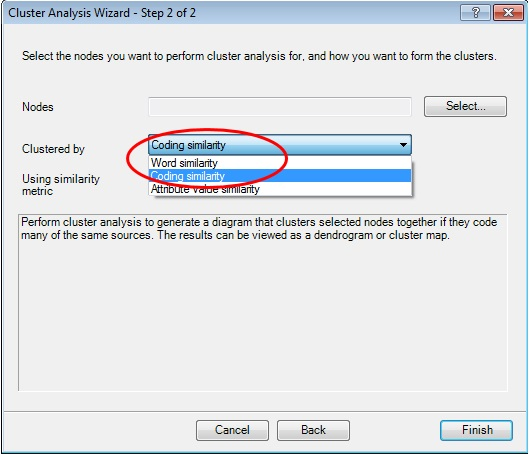Figure 9: Cluster analysis using coding similarity

After choosing ‘Coding Similarity’ as ‘Clustered by’ option select a ‘Similarity Metric’ (Figure 5). The case research uses ‘Correlation coefficient’ to perform cluster analysis. Click on ‘Finish’.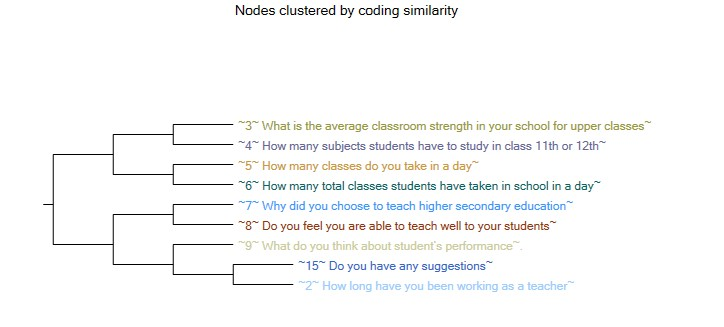Figure 10: Results of coding similarity in diagram view

## Interpretation of diagram view

To interpret diagram view export the diagram (figure above). In cluster analysis, nodes which are remotely connected are grouped together. Nodes 3, 4, 5 and 6 are answers to demographic questions in numerical form. Therefore they form one group. Similarly, answers of nodes 7,8,9,2 and 15 were present in text, thus contain maximum number of similar codes. To view the exact degree of coding similarity between these nodes, open summary view (figure below).Figure 11: Summary view

As mentioned above, manual coding hardly generates any common codes between the nodes. Summary view (figure above) represents the percentage of commonality between the codes. It turned out to be either zero or close to zero for every code. Therefore, coding similarity in the case research does not exist.

## Attribute value similarity

Cluster analysis through attribute value similarity is based on case nodes. Comparison takes place on attributes like demographic information. The case research uses cluster analysis of demographic information to compare case nodes. Some variables are school location, number of years or experience, class room strength. To perform attribute value similarity, follow these steps:

1. Click on dropdown of ‘Clustered by’
2. Select ‘Attribute value similarity’
3. Click on ‘Finish’ (figure below)Figure 12: Cluster analysis by attribute value similarity

The below figure represents the diagram view of this case research. As the figure shows, one group contains the responses of Preeti, Reshma, Kanwal and Rakhi. This is because they have similar attributes. Grouping of the responses of Neeraj, Reena, Namita and Natasha is also separate due to similar attributes among them.

The figure below shows the diagram view of these responses. Interview by Preeti, Reshma, Kanwal and Rakhi have maximum attribute similarities (figure below). Neeraj, Reena, Namita and Natasha have another set of similar attributes.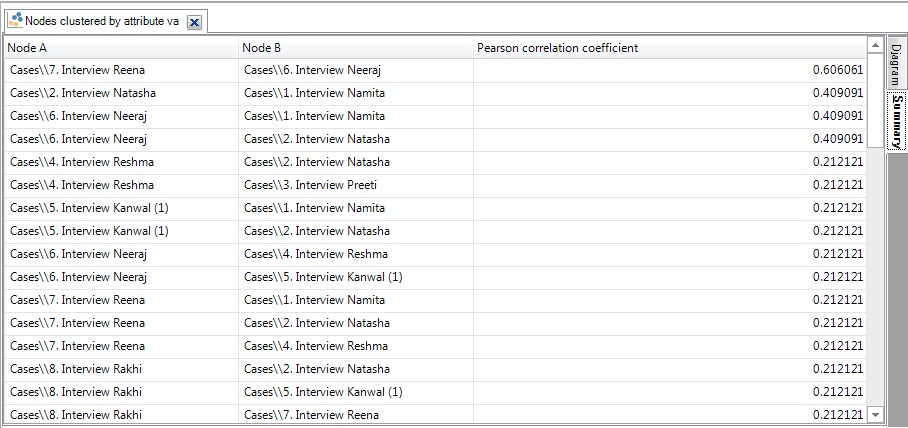Figure 14: Summary view of nodes clustered by attribute value similarity

Despite having similarities and groups, their degree of correlation is not significant. Reena and Neeraj exhibit highest correlation coefficient with greater than .50 value (.606). Correlation of remaining  groups is less than the acceptable value limit.

This article explained representation of results obtained from nodes using cluster analysis. Therefore the next article visualizes its results using mind maps. It shows a clear connection among nodes. Quality of education given to students of higher class students of selected schools of Delhi NCR can be seen using mind maps.

### Priya Chetty

Partner at Project Guru
Priya is a master in business administration with majors in marketing and finance. She is fluent with data modelling, time series analysis, various regression models, forecasting and interpretation of the data. She has assisted data scientists, corporates, scholars in the field of finance, banking, economics and marketing.We are looking for candidates who have completed their master's degree or Ph.D. Click here to know more about our vacancies.